# Excel TEXT function with formula examples

This tutorial explains the specificities of the Excel TEXT function and shows some clever ways to use Text formulas in Excel.

Microsoft Excel is all about numbers, and in your worksheets, you are likely to deal with numbers most of the time. However, there are situations when you need to convert numbers to text, and it is where the TEXT function steps in.

## Excel TEXT function - syntax and basic uses

In its pure essence, TEXT in Excel is used to convert a numeric value to a text string in a specific format.

The syntax for the Excel TEXT function is as follows:

TEXT(value, format_text)

Where:

• Value - the numeric value to be converted to text. It can be a number, date, reference to a cell containing a numeric value or another function that returns a number or date.
• Format_text - the format that you want to apply. It is supplied in the form of a format code enclosed in the quotation marks, e.g. "mm/dd/yy".

The TEXT function is available in all versions of Excel 2016, Excel 2013, Excel 2010, Excel 2007, Excel 2003, and lower.

Generally, an Excel TEXT formula is used in the following situations:

• To display numbers in a more readable way or in a format that makes more sense for your users.
• To display dates in a specific format.
• To combine numbers or dates with certain text or characters.

For example, if you want to pull the date from cell A2 and show it in another cell in the traditional date format like "January 1, 2016", you use the following Excel TEXT formula:

`=TEXT(A2, "mmmm d, yyyy")`

The result will look similar to this: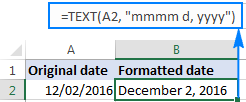Important note! The TEXT function in Excel converts a numeric value to a text strings. Consequently, you won't be able to use the result of your Text formula in other calculations. If there's such a need, you can keep the original values (hidden or kept out of sight), and use them in other formulas.

## Excel TEXT function format codes

As you have just seen, the syntax of the Excel TEXT function is very straightforward, a tricky part is supplying a proper format code that will output your number the way you want. Essentially, the TEXT function accepts most of the format codes used in Excel number formats. The table below lists the most common and frequently used ones.

 Code Description Format code example 0 Digit placeholder that displays insignificant zeros. #.00 - always displays 2 decimal places. If you type 2.5 in the referenced cell, it will display as 2.50. # Digit placeholder that does not display extra zeros. #.## - displays up to 2 decimal places. If you type 2.5, it will display as 2.5. If you type 2.555, it will display as 2.56. ? Digit placeholder that leaves a space for insignificant zeros but doesn't display them. It is generally used to align numbers in a column at a decimal point. #.?? - displays a maximum of 2 decimal places and aligns the decimal points in a column. . (period) Decimal point , (comma) Thousands separator. ###,###.## - displays a thousands separator and 2 decimal places. If you type 250000, it will display as 250,000.00

Additionally, you can include any of the following characters in the format code, and they will be displayed exactly as entered.

 Symbol Description + and - Plus and minus signs ( ) Left and right parenthesis : Colon ^ Caret ' Apostrophe { } Curly brackets < > Less-than and greater than signs = Equal sign / Forward slash ! Exclamation point & Ampersand ~ Tilde Space character

The following spreadsheet shows how you can use a Text formula in Excel to apply different formatting types to the same value.

A B C
1 Original Value Formatted Value Formula
2 5.5 5.50 =TEXT(A2, "0.00")
3   550% =TEXT(A2,"#%")
4   \$5.50 =TEXT(A2, "\$#,##0.00")
5   + \$5.50 =TEXT(A2, "+ \$#,##0.00;- \$#,##0.00;\$0.00")
6   - \$5.50 =TEXT(A2, "- \$#,##0.00;- \$#,##0.00;\$0.00")
7   5 1/2 =TEXT(A2,"# ?/?")
8   5.50E+00 =TEXT(A2,"0.00E+00")
9   ~6 ! =TEXT(A2,"~# !")

When using the Excel TEXT function with dates and times, you can use any of the following format codes.

 Format code Description Examples d Day of month, or day of week d - one or two-digit number without a leading zero (1 to 31) dd - two-digit number with a leading zero (01 to 31) ddd - three-letter abbreviation (Mon to Sun) dddd - full name of day of week (Monday to Sunday) m Month (when used as part of a date) m - one or two-digit number without a leading zero (1 to 12) mm - two-digit number with a leading zero (01 to 12) mmm - abbreviated month (Jan to Dec) mmmm - full name of month (January to December) y Year yy - two-digit number (e.g. 06 meaning 2006 or 16 meaning 2016) yyyy - four digit number (e.g. 2006, 2016) h Hour h - one or two-digit number without a leading zero (1 to 24) hh - two-digit number with a leading zero (01 to 24) m Minute (when used as part of time) m - one or two-digit number without a leading zero (1 to 60) mm - two-digit number with a leading zero (01 to 60) s Second s - one or two-digit number without a leading zero (1 to 60) ss - two-digit number with a leading zero (01 to 60) AM/PM Time represented as a 12-hour clock, followed by "AM" or "PM"

The following spreadsheet shows a few Excel Text formulas for dates:

A B C
1 Original Date Formatted Date Formula
2 12/09/2016 Dec 09 2016 =TEXT(A2, "mmm dd yyyy")
3   Friday 09 December, 2016 =TEXT(A2, "dddd dd mmmm, yyyy")
4   9-Dec-16 =TEXT(A2, "d-mmm-yy")
5   Friday =TEXT(A2,"dddd")

## How to use TEXT function in Excel - formula examples

So far, you might have an impression that the use of the Excel TEXT function is quite limited (because a value's display can easily be changed using the Format Cells dialog available via a cell's context menu or Ctrl+1 shortcut). The situation changes as soon as you start using TEXT in combination with other Excel functions. The below examples will give you a few inspirational ideas.

### Concatenate text and number (or date) in a custom format

When creating summary sheets or reports, you may often want not only calculate totals, percentages and the like, but also explain to your users what this or that number means. To do this, use the CONCATENATE function to join text and numbers, and the TEXT function to display the number (or date) like you want it.

#### Example 1. Format numbers inside a text string

Supposing you calculate the total amount based on the unit price in cell A2, quantity in B2, and discount percentage in C2 using this calculation: `=A2*B2*(1-C2)`. For your users to know exactly what the output number means, you wish to display it together with some explanatory text like "Your price is". Additionally, you want to show the currency symbol, thousands separator and two decimal places.

To have it done, supply the above calculation in the 1st argument of the TEXT function, include the corresponding format code in the 2nd argument, and concatenate the Text formula with a string using either the ampersand operator or CONCATENATE function:

`="Your price is "&TEXT(A2*B2*(1-C2), "\$###,###.00")`

or

`= CONCATENATE("Your price is ",TEXT(A2*B2*(1-C2), "\$###,###.00"))`

The following screenshot demonstrates the result: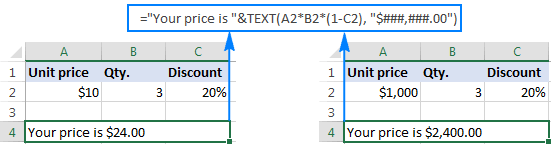#### Example 2. Combine text and date in the desired format

When you return the current date using TODAY() or NOW() function, you may also want to display it in combination with some text so that no one has any doubt what day this date refers to.

However, if you try to concatenate a text and date in the usual way:

`=CONCATENATE("Today is ", TODAY())`

Excel will return a very odd result, something like "Today is 42198".

The point is that in the internal Excel system, dates are stored as numbers, and that number appears in a concatenated text string. To fix this, use the TEXT function to display the date the way you want.

For example, embedding the TODAY function in the Text formula with the format code "dddd d mmm, yyyy" will return a string similar to this: "Today is Monday 12 Dec, 2016".

The complete formula goes as follows:

`=CONCATENATE("Today is ", TEXT(TODAY(), "dddd d mmm, yyyy"))`
or
`="Today is " & TEXT(TODAY(), "dddd d mmm, yyyy")`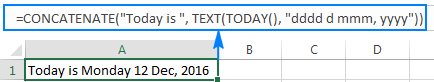A few more formula examples can be found here: Concatenate numbers and dates in various formats.

As you know, Microsoft Excel automatically removes leading zeros typed before a number in a cell, which works fine in most situations. But what if you want to keep the preceding zeros?

The Excel TEXT function can be an easy solution to pad numbers with leading zeros in a column, even if the original values are not the same length. Simply use the format code containing only zero placeholders like "0000000", where the number of zeros corresponds to the number of digits you want to display.

For example, to display 7-digit numbers with leading zeros, use this formula (where A2 is the original number):

`=TEXT(A2,"0000000")`

As you can see in the screenshot below, our Excel Text formula adds as many leading zeros as necessary to make a 7-character long string (please remember, the result of the TEXT function in Excel is always a text string, even if it looks like a number).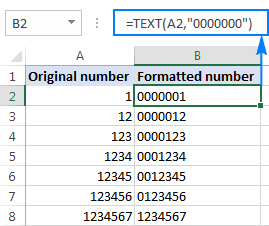### Convert values to phone numbers in a specific format

Turning a column of numeric values into telephone numbers may sound like a tricky task, but only until you remember that the Excel TEXT function allows using dashes and parentheses in format codes.

So, to display a number in A2 in a traditional US local 7-digit phone format like 123-456, use this formula:

`=TEXT(A2, "###-####")`

If some of the original values may contain a domestic prefix (i.e. there can be both 7-digit or 10-digit numbers), include the following conditional format code to display 10-digit numbers in the (123) 456-789 format:

`=TEXT(A2,"[<=9999999]###-####;(###) ###-####")`

The screenshot below shows this Excel Text formula in action: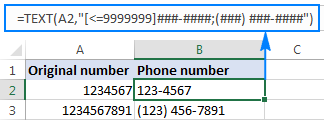## Excel TEXT function not working - reasons and solutions

Compared to other Excel functions, TEXT is very simple and painless, and you are unlikely to have any difficulties when using it in your spreadsheets. If, against all expectations, a Text formula is not working for you, in most cases it's because of an incorrect format code input in the format_text argument. Here are two most typical issues:

1. The TEXT function returns the #NAME? error if you omit the quotation marks around the format code.

For example the formula `=TEXT(A2, mm/dd/yy)` is incorrect and should be written this way: `=TEXT(A2, "mm/dd/yy")`

2. The TEXT function in Excel is language-specific, and requires using region-specific date and time format codes.

For example, the formula `=TEXT(A2, "mm/dd/yy")` that works fine for English users may return the #VALUE error in other locales. In particular, in Germany, you'd need to use the following format codes: "t" instead of "d" and "j" instead of "y" because "day" in German is "tag" and year is "jahr"; "m"(month) is fine because in German it also begins with "m" (monat). So, in German Excel, the above formula will read as follows: `=TEXT(A2; "mm/tt/jj")`. In France, you'd use the "mm/jj/aa" format code because "day" is "jour", "month" is "mois", and "year" is "an".

Also, please pay attention that in European countries, the List Separator is usually set to semicolon, not comma, and therefore ";" should be used to separate the formula's arguments.

This is how you use TEXT in Excel. If someone wants to have a closer look at the formulas discussed in this tutorial, you are welcome to download the Sample Excel TEXT function worksheet.

## Other Excel Text functions

Apart from TEXT, there are a handful of other functions to work with text values in Excel. Some of them are designed to convert text into numbers and the other way round, while others are used for manipulating text strings themselves. Below you will find formula examples of both types.

### 38 Responses to "Excel TEXT function with formula examples"

1.luke says:

i am concatenating headings and associated numbers for a report.
all of the numbers have a currency fomrat.
i am using the followning function to show thousand separators: "£#,##0;;@"
the positiveappear fine but the negetive numbers do not appear

•Svetlana Cheusheva (Ablebits.com Team) says:

Hi Luke,

This is because the negative numbers section is empty in your format code.
To have the format apply to all number types - positive, negative and zeros - use only the first section of the format code: "£#,##0"

2.Piet says:

Hi,
I would like to know how I can add text to the number format?
Usually, if you edit the cell format, I would enter a custom format:

dd "Days" hh "Hours"

But if I try that in the TEXT function, it doesn't work because of the extra " characters:

=text(today(), "dd "Days" hh "Hours"")

Any idea of a work around?

•Simon says:

Hi Piet,
Does using =TEXT(TODAY(),"dd ") & "Days " & TEXT(TODAY(),"hh ") & "Hours" give you what you require?

3.Rajesh Sinha says:

My question is,, in this formula =if(A1>B1, "Yes", "No").
I want Yes in bold, is it possible using Text function.

•Svetlana Cheusheva (Ablebits.com Team) says:

Hello Rajesh,

The TEXT function can only change the way a number appears in a cell by applying a custom number format to it. It cannot apply bold, italic, or underlining style. To my best knowledge, none of the Excel functions can do that.

•Chan says:

You can apply Conditional Formatting instead. It will be easier.

4.Ahmad OUMARA says:

=TEXT(A1;"FL-00;BS-00;GR±00;@")
hello, iam trying to use the mentioned function to add prefix (FL-) before any positive number or (BS-) before any negative number or (GR±00) if the number is 0 or to use the same text in cell

this function is working for positive numbers only and for zero it shows (R±00) without G .

if you can help me, I will be appreciated

•Natalia Sharashova (Ablebits.com Team) says:

Hello,

=TEXT(A1;"\F\L\-00;\B\S\-00;\G\R±00; @")

Hope it helps!

5.Jim L says:

I am wanting to split dates into 'Month' A and 'Month' B where up to the 15th is A and 16th onwards is B.
With the Date in cell A1, I have tried both these formulas
=TEXT(A1,IF(DAY(A1)<=15,"Mmm YY A","Mmm YY B"))
=TEXT(A1,IF(DAY(A1)<=15,"Mmm YY A",CONCATENATE("Mmm YY"," B")))
If the date is 15th or below it shows A correctly but otherwise on the first I get #VALUE! and on the second instead of B, it shows 60, i.e. Nov 17 60.

I'm sure there must be a way but I can't find it

6.Jim L says:

Sorry I should have looked at the question above before posting mine. It is:
=TEXT(A1,IF(DAY(A1)<=15,"Mmm YY \A","Mmm YY \B"))

7.Wan says:

In the formula below, the negative difference does not appear in Red. Is there a different formatting method to make the negatives red?

=TEXT(+H37-G37,"#,##0_);[RED](#,##0)")&" ( "&TEXT(IFERROR(H37/G37-1,0),"%0.0")&" )"

•Gennady Terekhov (Ablebits.com Team) says:

Hello,

Please note that the TEXT function does not support color formatting. So if you copy a number format code from the Format Cells dialog that includes a color, e. g. \$#,##0.00_);[Red](\$#,##0.00), the TEXT function will accept the format code, but it won’t display the color.

8.Sanobar khan says:

Hi Dear,
please tell me the way of writing in Excel 2010 like this
0001-FFFSL-1-17. now i want to change the first number in this not change the last by pressing Ctrl+Mouse Scroling. as the following is needing 0001-FFFSL-1-17 0002-FFFSL-1-17 0003-FFFSL-1-17

9.lori says:

I am using text formula to display other cells as a certain amount of digits with leading zeroes. all is well with that. what I am unable to figure out is how to get a blank in my result when the original cell is blank. right now, with the basic formula, it displays all zeroes in the amount of digits I've chosen, but as I'm saying, I want to return a blank cell. I've tried =if(isblank)etc with the text formula embedded if the cell is not blank (hope that makes sense) but that either doesn't work or I've gotten the syntax wrong. can anyone help me here?? thank you *SO* much for any help anyone can give me!

10.Joshua says:

I have one:

=Sum(G36-D6)/8)& " Days"

and its returning a xx.xxxx
I only want it to return a xx.xx

11.Claudio says:

Hi,
Could someone help me with the formula that when type a code it shows the text from another cell, please?
I need to make it easier when I'm requesting supplies from my stock excel file just typing the code of the product.

Thanks

12.pete says:

Hi

Today is 1 Feb 2018 (01/02/2018 in dd/mm/yyyy).

I want to retrieve the month portion of the current date.

I can do that with MONTH(TODAY()), and it will return 2.

So far so good.

If I try TEXT(MONTH(TODAY()),"mm"), it returns "01".

I have tried using a new sheet on my Citrix computer - it returns "01".

I have tried using a new sheet on my BBB Android phone it returns "01".

Any clues?

Pete
(BBB = Beloved BlackBerry)

•Peter says:

Hi Pete,
You're getting that problem because you're converting the date to the number 2, and then you're telling it to convert the number 2 into a date, which it then knows as 02/01/1900 (dd/mm/yyyy), so then when you run the text formula, you're getting the 1 from the January.

13.Slee says:

I am trying to get the formula ="+ "&SUM(I29-D29)/D29 to appear as +23% It appears as +0.231952922620801 Have tried clicking % function button, using formula pull down menu and format menu. Nothing works. Is it possible to place a text + in front of a calculated percentage in excel? Yes, I realize the plus is assumed but my boss wants it to appear in the spreadsheet.

Using I29 number of 246,823
D29 number as 200,351

•Jeremy says:

ROUND the number you're inserting into the text field and multiply by 100
ie &ROUND(SUM(I29-D290)/D29,2)*100 will convert the 0.23195... into 0.23 * 100 = 23

14.Oloyede Abdulsalam says:

I created a new column and name it month so I want the entire column to be filled with months I.e January to december how can I do it and which formula can I use

15.Sti says:

•Doug says:

Sti:
I believe you can get the formatting you want by right clicking the cell that holds the data then Format Cells then Custom then enter .0000 in the Type field and OK.

•Svetlana Cheusheva (Ablebits.com Team) says:

In addition to Doug's response (again, thank you Doug!):

If your values have a variable number of digits after the decimal point, you may want to use the # placeholder that does not display extra zeros.

For example, the .0000 format code will display 0.183 as .1830 and the .#### format code will display it as .183

16.nick says:

Hi,

i want result in bold with using CONCATENATE without using VBA code.

For eg 1. Paneer 2. Egg

So Result should be in =CONCATENATE (Paneer Egg(in Bold))

can any one help me

17.Shankar says:

I put CONCATENATE formula in cell (B10) with four different cell value from cell (N5), (N6), (N7) & (N8). I want to make Bold for all the text shown from Cell, N5, N6, N7 & N8 in my Concatenate formula.

Can any one help...

18.kanil says:

I would like to display the Custom Number format used
in a new cell just like TEXTFORMULA function displays the FORMULA
S0 since I have a bad memeory 80 yrs old, I can then see the previous work and immediately know the Custom Number format that was asssociated with it, which is now displayed adjacent to it

This will help others (learning) when worksheet is shared -
Thanks in advance - Fragranace Of The Universe Is Gratitude"

19.Jagjeet Das says:

The___________character is used in text formula.

20.Walt says:

I'm trying to concatenate a number with the " (double quote) sign.

So I'd like to convert the number 90 to 90"

I've tried =text(A1,"""), where the number is in A1, but no luck. Any ideas?

•Jeremy says:

Try putting the " outside the text conversion =text(A1)&" or even just =A1&"""

21.Ariel says:

Hi i am trying to create a string of numbers with initials at the end that is populated from a cell with an if statement.
i need the number to read 2019-000-AA
2019 comes from cell L12, 000 is from cell M12 (i need the leading Zero to show when the number is only a double digit or single digit - i need it to have the 0 placeholders) and AA is from J4.
I also have a macro that needs to run adding plus 1 to the number in cell M12. i have the code for this, but it is not working and cell I4 when =L12&M12&J4 does not show the 0 place holder before the middle number (M12)

22.Kumar says:

Hello,
I need formula where in column A with percentage changing against text which is having in column B.
Ewample : Column A = 0%, 25%, 75%, 85% and 100%
Coumn B = WIP, complete, Ready, AWIP, delivered
how can I write it.
Regards,
Kumar

23.sami says:

my question is =if(a1>=250rgb,"a2*60")

24.melissa says:

how would i convert amount into words

25.Kevin says:

Hi,
How can I format a function results to "underline" part of the string??

Ex: I need to calculate todays date and print "Today is: 8/31/2019" WITH ONLY "8/31/2019" UNDERSCORED.

26.Ashraf83 says:

Hi
I would like to convert number from currency to text
How can i do this, my number format let say for example RM34.00 so the bank want it to be in 00000340000 11 digits, how to do it
I got atleast 2k data

27.Sharon says:

When I import a text field "1E1" into MS Excel, it converts to "1.00E+01". How can I stop it from converting OR what is a formula to reverse the scientific notation. Currently, when I try to convert it back it turns into "10".

60+ professional tools for Excel